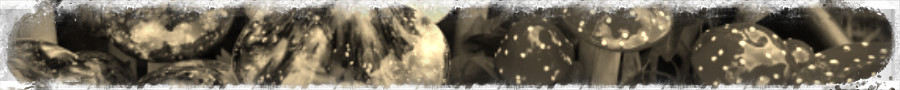# Introduction

This vignette gives you a quick introduction to data.tree applications. We took care to keep the examples simple enough so non-specialists can follow them. The price for this is, obviously, that the examples are often simple compared to real-life applications.

If you are using data.tree for things not listed here, and if you believe this is of general interest, then please do drop us a note, so we can include your application in a future version of this vignette.

# World PopulationTreeMap (visualization)

This example is inspired by the examples of the treemap package.

You’ll learn how to

• convert a data.frame to a data.tree structure
• navigate a tree and locate specific nodes
• use Aggregate and Cumulate
• manipulate an existing tree, e.g. by using the Prune method

## Original Example, to be improved

The original example visualizes the world population as a tree map.

library(treemap)
data(GNI2014)
treemap(GNI2014,
index=c("continent", "iso3"),
vSize="population",
vColor="GNI",
type="value")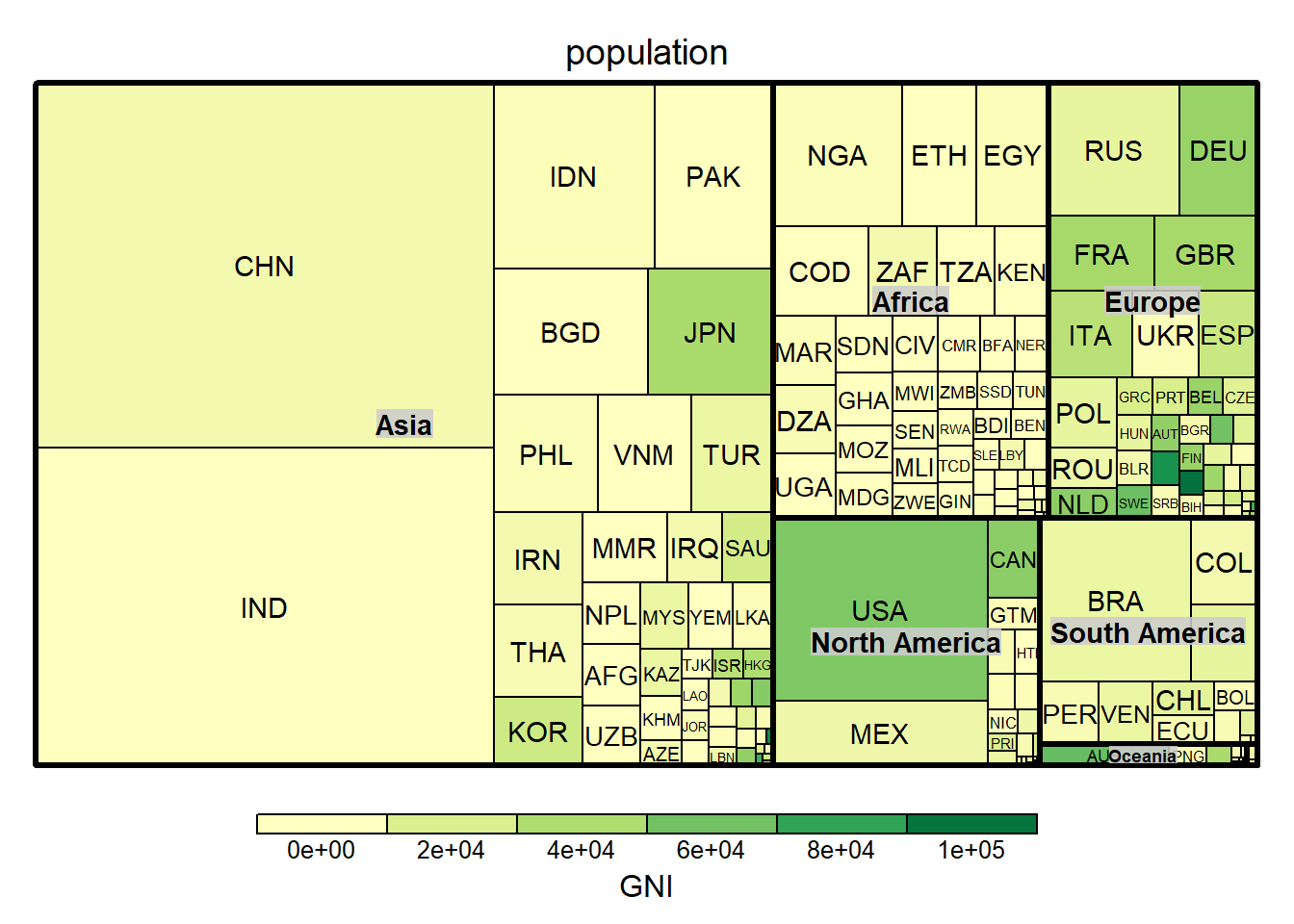As there are many countries, the chart gets clustered with many very small boxes. In this example, we will limit the number of countries and sum the remaining population in a catch-all country called “Other”.

We use data.tree to do this aggregation.

## Convert from data.frame

First, let’s convert the population data into a data.tree structure:

library(data.tree)
GNI2014$continent <- as.character(GNI2014$continent)
GNI2014$pathString <- paste("world", GNI2014$continent, GNI2014$country, sep = "/") tree <- as.Node(GNI2014[,]) print(tree, pruneMethod = "dist", limit = 20) ## levelName ## 1 world ## 2 ¦--North America ## 3 ¦ ¦--Bermuda ## 4 ¦ ¦--United States ## 5 ¦ °--... 22 nodes w/ 0 sub ## 6 ¦--Europe ## 7 ¦ ¦--Norway ## 8 ¦ ¦--Switzerland ## 9 ¦ °--... 39 nodes w/ 0 sub ## 10 ¦--Asia ## 11 ¦ ¦--Qatar ## 12 ¦ ¦--Macao SAR, China ## 13 ¦ °--... 45 nodes w/ 0 sub ## 14 ¦--Oceania ## 15 ¦ ¦--Australia ## 16 ¦ ¦--New Zealand ## 17 ¦ °--... 11 nodes w/ 0 sub ## 18 ¦--South America ## 19 ¦ ¦--Uruguay ## 20 ¦ ¦--Chile ## 21 ¦ °--... 10 nodes w/ 0 sub ## 22 ¦--Seven seas (open ocean) ## 23 ¦ ¦--Seychelles ## 24 ¦ ¦--Mauritius ## 25 ¦ °--... 1 nodes w/ 0 sub ## 26 °--Africa ## 27 °--... 48 nodes w/ 0 sub We can also navigate the tree to find the population of a specific country. Luckily, RStudio is quite helpful with its code completion (use CTRL + SPACE): tree$Europe$Switzerland$population
##  7604467

Or, we can look at a sub-tree:

northAm <- tree$North America Sort(northAm, "GNI", decreasing = TRUE) print(northAm, "iso3", "population", "GNI", limit = 12) ## levelName iso3 population GNI ## 1 North America NA NA ## 2 ¦--Bermuda BMU 67837 106140 ## 3 ¦--United States USA 313973000 55200 ## 4 ¦--Canada CAN 33487208 51630 ## 5 ¦--Bahamas, The BHS 309156 20980 ## 6 ¦--Trinidad and Tobago TTO 1310000 20070 ## 7 ¦--Puerto Rico PRI 3971020 19310 ## 8 ¦--Barbados BRB 284589 15310 ## 9 ¦--St. Kitts and Nevis KNA 40131 14920 ## 10 ¦--Antigua and Barbuda ATG 85632 13300 ## 11 ¦--Panama PAN 3360474 11130 ## 12 °--... 14 nodes w/ 0 sub NA NA Or, we can find out what is the country with the largest GNI: maxGNI <- Aggregate(tree, "GNI", max) #same thing, in a more traditional way: maxGNI <- max(sapply(tree$leaves, function(x) x$GNI)) tree$Get("name", filterFun = function(x) x$isLeaf && x$GNI == maxGNI)
##   Bermuda
## "Bermuda"

## Aggregate and Cumulate

We aggregate the population. For non-leaves, this will recursively iterate through children, and cache the result in the population field.

tree$Do(function(x) { x$population <- Aggregate(node = x,
attribute = "population",
aggFun = sum)
},
traversal = "post-order")

Next, we sort each node by population:

Sort(tree, attribute = "population", decreasing = TRUE, recursive = TRUE)

Finally, we cumulate among siblings, and store the running sum in an attribute called cumPop:

tree$Do(function(x) x$cumPop <- Cumulate(x, "population", sum))

The tree now looks like this:

print(tree, "population", "cumPop", pruneMethod = "dist", limit = 20)
##                        levelName population     cumPop
## 1  world                         6683146875 6683146875
## 2   ¦--Asia                      4033277009 4033277009
## 3   ¦   ¦--China                 1338612970 1338612970
## 4   ¦   ¦--India                 1166079220 2504692190
## 5   ¦   °--... 45 nodes w/ 0 sub         NA         NA
## 6   ¦--Africa                     962382035 4995659044
## 7   ¦   ¦--Nigeria                149229090  149229090
## 8   ¦   ¦--Ethiopia                85237338  234466428
## 9   ¦   °--... 46 nodes w/ 0 sub         NA         NA
## 10  ¦--Europe                     728669949 5724328993
## 11  ¦   ¦--Russian Federation     140041247  140041247
## 12  ¦   ¦--Germany                 82329758  222371005
## 13  ¦   °--... 39 nodes w/ 0 sub         NA         NA
## 14  ¦--North America              528748158 6253077151
## 15  ¦   ¦--United States          313973000  313973000
## 16  ¦   ¦--Mexico                 111211789  425184789
## 17  ¦   °--... 22 nodes w/ 0 sub         NA         NA
## 18  ¦--South America              394352338 6647429489
## 19  ¦   ¦--Brazil                 198739269  198739269
## 20  ¦   ¦--Colombia                45644023  244383292
## 21  ¦   °--... 10 nodes w/ 0 sub         NA         NA
## 22  ¦--Oceania                     33949312 6681378801
## 23  ¦   ¦--Australia               21262641   21262641
## 24  ¦   ¦--Papua New Guinea         6057263   27319904
## 25  ¦   °--... 11 nodes w/ 0 sub         NA         NA
## 26  °--Seven seas (open ocean)      1768074 6683146875
## 27      °--... 3 nodes w/ 0 sub          NA         NA

## Prune

The previous steps were done to define our threshold: big countries should be displayed, while small ones should be grouped together. This lets us define a pruning function that will allow a maximum of 7 countries per continent, and that will prune all countries making up less than 90% of a continent’s population.

We would like to store the original number of countries for further use:

tree$Do(function(x) x$origCount <- x$count) We are now ready to prune. This is done by defining a pruning function, returning ‘FALSE’ for all countries that should be combined: myPruneFun <- function(x, cutoff = 0.9, maxCountries = 7) { if (isNotLeaf(x)) return (TRUE) if (x$position > maxCountries) return (FALSE)
return (x$cumPop < (x$parent$population * cutoff)) } We clone the tree, because we might want to play around with different parameters: treeClone <- Clone(tree, pruneFun = myPruneFun) print(treeClone$Oceania, "population", pruneMethod = "simple", limit = 20)
##              levelName population
## 1 Oceania                33949312
## 2  ¦--Australia          21262641
## 3  °--Papua New Guinea    6057263

Finally, we need to sum countries that we pruned away into a new “Other” node:

treeClone$Do(function(x) { missing <- x$population - sum(sapply(x$children, function(x) x$population))
other <- x$AddChild("Other") other$iso3 <- paste0("OTH(", x$origCount, ")") other$country <- "Other"
other$continent <- x$name
other$GNI <- 0 other$population <- missing
},
filterFun = function(x) x$level == 2 ) print(treeClone$Oceania, "population", pruneMethod = "simple", limit = 20)
##              levelName population
## 1 Oceania                33949312
## 2  ¦--Australia          21262641
## 3  ¦--Papua New Guinea    6057263
## 4  °--Other               6629408

## Plot

### Plotting the treemap

In order to plot the treemap, we need to convert the data.tree structure back to a data.frame:

df <- ToDataFrameTable(treeClone, "iso3", "country", "continent", "population", "GNI")

treemap(df,
index=c("continent", "iso3"),
vSize="population",
vColor="GNI",
type="value")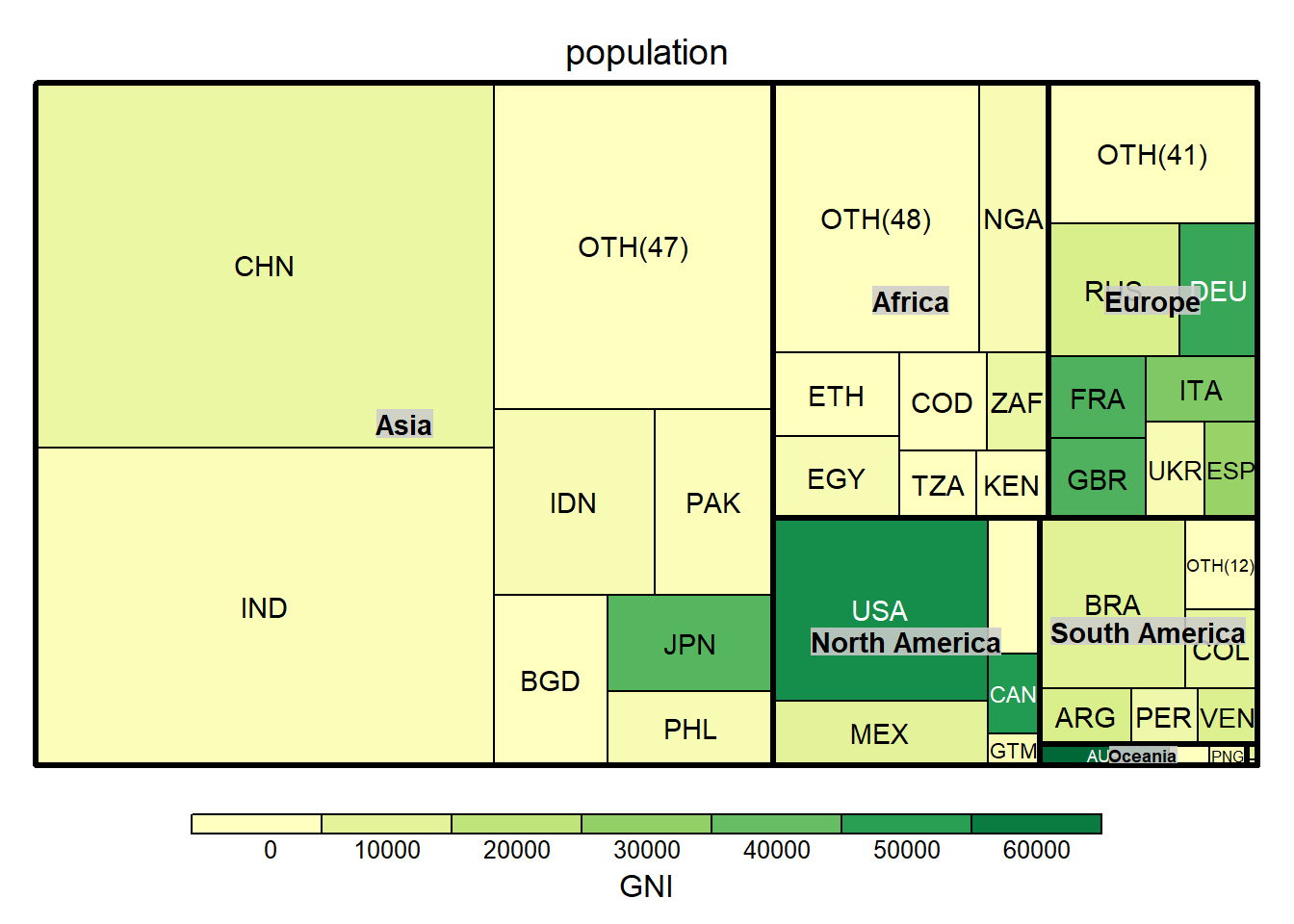### Plot as dendrogram

Just for fun, and for no reason other than to demonstrate conversion to dendrogram, we can plot this in a very unusual way:

plot(as.dendrogram(treeClone, heightAttribute = "population"))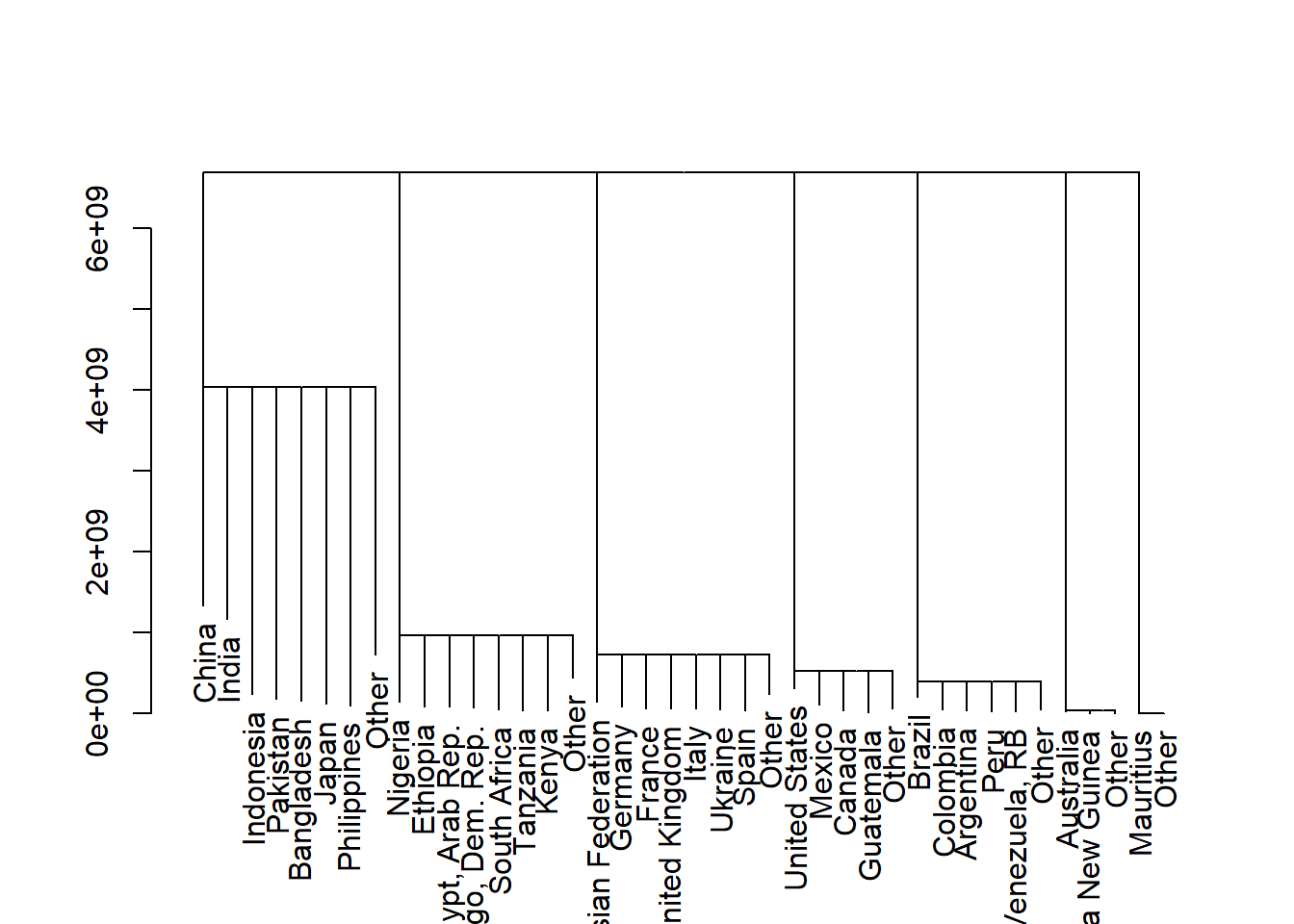## Further developments

Obviously, we should also aggregate the GNI as a weighted average. Namely, we should do this for the OTH catch-all countries that we add to the tree.

# Portfolio Breakdown (finance)

In this example, we show how to display an investment portfolio as a hierarchic breakdown into asset classes. You’ll see:

• how you can re-use a traversal
• advanced use of Aggregate

## Convert from data.frame

fileName <- system.file("extdata", "portfolio.csv", package="data.tree")
pfodf <- read.csv(fileName, stringsAsFactors = FALSE)
head(pfodf)
##           ISIN                                     Name Ccy Type Duration
## 1 LI0015327682          LGT Money Market Fund (CHF) - B CHF Fund       NA
## 2 LI0214880598        CS (Lie) Money Market Fund EUR EB EUR Fund       NA
## 3 LI0214880689        CS (Lie) Money Market Fund USD EB USD Fund       NA
## 4 LU0243957825    Invesco Euro Corporate Bond A EUR Acc EUR Fund     5.10
## 5 LU0408877412 JPM Euro Gov Sh. Duration Bd A (acc)-EUR EUR Fund     2.45
## 6 LU0376989207 Aberdeen Global Sel Emerg Mkt Bd A2 HEUR EUR Fund     6.80
##   Weight AssetCategory AssetClass        SubAssetClass
## 1  0.030          Cash        CHF
## 2  0.060          Cash        EUR
## 3  0.020          Cash        USD
## 4  0.120  Fixed Income        EUR Sov. and Corp. Bonds
## 5  0.065  Fixed Income        EUR Sov. and Corp. Bonds
## 6  0.030  Fixed Income        EUR       Em. Mkts Bonds

Let us convert the data.frame to a data.tree structure. Here, we use again the path string method. For other options, see ?as.Node.data.frame

pfodf$pathString <- paste("portfolio", pfodf$AssetCategory,
pfodf$AssetClass, pfodf$SubAssetClass,
pfodf$ISIN, sep = "/") pfo <- as.Node(pfodf) ## Aggregate To calculate the weight per asset class, we use the Aggregate method: t <- Traverse(pfo, traversal = "post-order") Do(t, function(x) x$Weight <- Aggregate(node = x, attribute = "Weight", aggFun = sum))

We now calculate the WeightOfParent,

Do(t, function(x) x$WeightOfParent <- x$Weight / x$parent$Weight)

Duration is a bit more complicated, as this is a concept that applies only to the fixed income asset class. Note that, in the second statement, we are reusing the traversal from above.

pfo$Do(function(x) x$Duration <- ifelse(is.null(x$Duration), 0, x$Duration), filterFun = isLeaf)
Do(t, function(x) x$Duration <- Aggregate(x, function(x) x$WeightOfParent * x$Duration, sum)) ## Formatters We can add default formatters to our data.tree structure. Here, we add them to the root, but we might as well add them to any Node in the tree. SetFormat(pfo, "WeightOfParent", function(x) FormatPercent(x, digits = 1)) SetFormat(pfo, "Weight", FormatPercent) FormatDuration <- function(x) { if (x != 0) res <- FormatFixedDecimal(x, digits = 1) else res <- "" return (res) } SetFormat(pfo, "Duration", FormatDuration) These formatter functions will be used when printing a data.tree structure. ## Print #Print print(pfo, "Weight", "WeightOfParent", "Duration", filterFun = function(x) !x$isLeaf)
##                           levelName   Weight WeightOfParent Duration
## 1  portfolio                        100.00 %                     0.8
## 2   ¦--Cash                          11.00 %         11.0 %
## 3   ¦   ¦--CHF                        3.00 %         27.3 %
## 4   ¦   ¦--EUR                        6.00 %         54.5 %
## 5   ¦   °--USD                        2.00 %         18.2 %
## 6   ¦--Fixed Income                  28.50 %         28.5 %      3.0
## 7   ¦   ¦--EUR                       26.00 %         91.2 %      3.1
## 8   ¦   ¦   ¦--Sov. and Corp. Bonds  18.50 %         71.2 %      2.4
## 9   ¦   ¦   ¦--Em. Mkts Bonds         3.00 %         11.5 %      6.8
## 10  ¦   ¦   °--High Yield Bonds       4.50 %         17.3 %      3.4
## 11  ¦   °--USD                        2.50 %          8.8 %      1.6
## 12  ¦       °--High Yield Bonds       2.50 %        100.0 %      1.6
## 13  ¦--Equities                      40.00 %         40.0 %
## 14  ¦   ¦--Switzerland                6.00 %         15.0 %
## 15  ¦   ¦--Euroland                  14.50 %         36.2 %
## 16  ¦   ¦--US                         8.10 %         20.2 %
## 17  ¦   ¦--UK                         0.90 %          2.2 %
## 18  ¦   ¦--Japan                      3.00 %          7.5 %
## 19  ¦   ¦--Australia                  2.00 %          5.0 %
## 20  ¦   °--Emerging Markets           5.50 %         13.7 %
## 21  °--Alternative Investments       20.50 %         20.5 %
## 22      ¦--Real Estate                5.50 %         26.8 %
## 23      ¦   °--Eurozone               5.50 %        100.0 %
## 24      ¦--Hedge Funds               10.50 %         51.2 %
## 25      °--Commodities                4.50 %         22.0 %

# ID3 (machine learning)

This example shows you the following:

• How to build a data.tree structure in an algorithm
• How to prune a tree
• How to use data.tree to develop learning algorithms

Classification trees are very popular these days. If you have never come across them, you might be interested in classification trees. These models let you classify observations (e.g. things, outcomes) according to the observations’ qualities, called features. Essentially, all of these models consist of creating a tree, where each node acts as a router. You insert your mushroom instance at the root of the tree, and then, depending on the mushroom’s features (size, points, color, etc.), you follow along a different path, until a leaf node spits out your mushroom’s class, i.e. whether it’s edible or not.

There are two different steps involved in using such a model: training (i.e. constructing the tree), and predicting (i.e. using the tree to predict whether a given mushroom is poisonous). This example provides code to do both, using one of the very early algorithms to classify data according to discrete features: ID3. It lends itself well for this example, but of course today there are much more elaborate and refined algorithms available.

## ID3 Introduction

During the prediction step, each node routes our mushroom according to a feature. But how do we chose the feature? Should we first separate our set according to color or size? That is where classification models differ.

In ID3, we pick, at each node, the feature with the highest Information Gain. In a nutshell, this is the feature which splits the sample in the possibly purest subsets. For example, in the case of mushrooms, dots might be a more sensible feature than organic.

### Purity and Entropy

IsPure <- function(data) {
length(unique(data[,ncol(data)])) == 1
}

The entropy is a measure of the purity of a dataset.

Entropy <- function( vls ) {
res <- vls/sum(vls) * log2(vls/sum(vls))
res[vls == 0] <- 0
-sum(res)
}

### Information Gain

Mathematically, the information gain IG is defined as:

$IG(T,a) = H(T)-\sum_{v\in vals(a)}\frac{|\{\textbf{x}\in T|x_a=v\}|}{|T|} \cdot H(\{\textbf{x}\in T|x_a=v\})$

In words, the information gain measures the difference between the entropy before the split, and the weighted sum of the entropies after the split.

So, let’s rewrite that in R:

InformationGain <- function( tble ) {
entropyBefore <- Entropy(colSums(tble))
s <- rowSums(tble)
entropyAfter <- sum (s / sum(s) * apply(tble, MARGIN = 1, FUN = Entropy ))
informationGain <- entropyBefore - entropyAfter
return (informationGain)
}

## Training

We are all set for the ID3 training algorithm.

### Pseudo code

We start with the entire training data, and with a root. Then:

1. if the data-set is pure (e.g. all toxic), then
1. construct a leaf having the name of the class (e.g. ‘toxic’)
2. else
1. choose the feature with the highest information gain (e.g. ‘color’)
2. for each value of that feature (e.g. ‘red’, ‘brown’, ‘green’)
1. take the subset of the data-set having that feature value
2. construct a child node having the name of that feature value (e.g. ‘red’)
3. call the algorithm recursively on the child node and the subset

### Implementation in R with the data.tree package

For the following implementation, we assume that the classifying features are in columns 1 to n-1, whereas the class (the edibility) is in the last column.

TrainID3 <- function(node, data) {

node$obsCount <- nrow(data) #if the data-set is pure (e.g. all toxic), then if (IsPure(data)) { #construct a leaf having the name of the pure feature (e.g. 'toxic') child <- node$AddChild(unique(data[,ncol(data)]))
node$feature <- tail(names(data), 1) child$obsCount <- nrow(data)
child$feature <- '' } else { #calculate the information gain ig <- sapply(colnames(data)[-ncol(data)], function(x) InformationGain( table(data[,x], data[,ncol(data)]) ) ) #chose the feature with the highest information gain (e.g. 'color') #if more than one feature have the same information gain, then take #the first one feature <- names(which.max(ig)) node$feature <- feature

#take the subset of the data-set having that feature value

childObs <- split(data[ ,names(data) != feature, drop = FALSE],
data[ ,feature],
drop = TRUE)

for(i in 1:length(childObs)) {
#construct a child having the name of that feature value (e.g. 'red')
child <- node$AddChild(names(childObs)[i]) #call the algorithm recursively on the child and the subset TrainID3(child, childObs[[i]]) } } } ### Training with data Our training data looks like this: library(data.tree) data(mushroom) mushroom ## color size points edibility ## 1 red small yes toxic ## 2 brown small no edible ## 3 brown large yes edible ## 4 green small no edible ## 5 red large no edible Indeed, a bit small. But you get the idea. We are ready to train our decision tree by running the function: tree <- Node$new("mushroom")
TrainID3(tree, mushroom)
print(tree, "feature", "obsCount")
##             levelName   feature obsCount
## 1  mushroom               color        5
## 2   ¦--brown          edibility        2
## 3   ¦   °--edible                      2
## 4   ¦--green          edibility        1
## 5   ¦   °--edible                      1
## 6   °--red                 size        2
## 7       ¦--large      edibility        1
## 8       ¦   °--edible                  1
## 9       °--small      edibility        1
## 10          °--toxic                   1

## Prediction

### The prediction method

We need a predict function, which will route data through our tree and make a prediction based on the leave where it ends up:

Predict <- function(tree, features) {
if (tree$children[]$isLeaf) return (tree$children[]$name)
child <- tree$children[[features[[tree$feature]]]]
return ( Predict(child, features))
}

### Using the prediction method

And now we use it to predict:

Predict(tree, c(color = 'red',
size = 'large',
points = 'yes')
)
##  "edible"

Oops! Looks like trusting classification blindly might get you killed.

# Jenny Lind (decision tree, plotting)

This demo calculates and plots a simple decision tree. It demonstrates the following:

• how to read a yaml file into a data.tree structure
• how to calculate a decision tree
• how to plot a data.tree with the data.tree plotting facility

YAML is similar to JSON, but targeted towards humans (as opposed to computers). It’s consise and easy to read. YAML can be a neat format to store your data.tree structures, as you can use it across different software and systems, you can edit it with any text editor, and you can even send it as an email.

This is how our YAML file looks:

fileName <- system.file("extdata", "jennylind.yaml", package="data.tree")
cat(readChar(fileName, file.info(fileName)$size)) ## name: Jenny Lind ## type: decision ## Sign with Movie Company: ## type: chance ## Small Box Office: ## type: terminal ## p: 0.3 ## payoff: 200000 ## Medium Box Office: ## type: terminal ## p: 0.6 ## payoff: 1000000 ## Large Box Office: ## type: terminal ## p: 0.1 ## payoff: 3000000 ## Sign with TV Network: ## type: chance ## Small Box Office: ## type: terminal ## p: 0.3 ## payoff: 900000 ## Medium Box Office: ## type: terminal ## p: 0.6 ## payoff: 900000 ## Large Box Office: ## type: terminal ## p: 0.1 ## payoff: 900000 Let’s convert the YAML into a data.tree structure. First, we load it with the yaml package into a list of lists. Then we use as.Node to convert the list into a data.tree structure: library(data.tree) library(yaml) lol <- yaml.load_file(fileName) jl <- as.Node(lol) print(jl, "type", "payoff", "p") ## levelName type payoff p ## 1 Jenny Lind decision NA NA ## 2 ¦--Sign with Movie Company chance NA NA ## 3 ¦ ¦--Small Box Office terminal 200000 0.3 ## 4 ¦ ¦--Medium Box Office terminal 1000000 0.6 ## 5 ¦ °--Large Box Office terminal 3000000 0.1 ## 6 °--Sign with TV Network chance NA NA ## 7 ¦--Small Box Office terminal 900000 0.3 ## 8 ¦--Medium Box Office terminal 900000 0.6 ## 9 °--Large Box Office terminal 900000 0.1 ## Calculate Next, we define our payoff function, and apply it to the tree. Note that we use post-order traversal, meaning that we calculate the tree from leaf to root: payoff <- function(node) { if (node$type == 'chance') node$payoff <- sum(sapply(node$children, function(child) child$payoff * child$p))
else if (node$type == 'decision') node$payoff <- max(sapply(node$children, function(child) child$payoff))
}

jl$Do(payoff, traversal = "post-order", filterFun = isNotLeaf) The decision function is the next step. Note that we filter on decision nodes: decision <- function(x) { po <- sapply(x$children, function(child) child$payoff) x$decision <- names(po[po == x$payoff]) } jl$Do(decision, filterFun = function(x) x$type == 'decision') ## Plot ### Plot with the data.tree plotting facility The data tree plotting facility uses GraphViz / DiagrammeR. You can provide a function as a style: GetNodeLabel <- function(node) switch(node$type,
terminal = paste0( '$', format(node$payoff, scientific = FALSE, big.mark = ",")),
paste0('ER\n', '$', format(node$payoff, scientific = FALSE, big.mark = ",")))

GetEdgeLabel <- function(node) {
if (!node$isRoot && node$parent$type == 'chance') { label = paste0(node$name, " (", node$p, ")") } else { label = node$name
}
return (label)
}

GetNodeShape <- function(node) switch(node$type, decision = "box", chance = "circle", terminal = "none") SetEdgeStyle(jl, fontname = 'helvetica', label = GetEdgeLabel) SetNodeStyle(jl, fontname = 'helvetica', label = GetNodeLabel, shape = GetNodeShape) Note that the fontname is inherited as is by all children, whereas e.g. the label argument is a function, it’s called on each inheriting child node. Another alternative is to set the style per node: jl$Do(function(x) SetEdgeStyle(x, color = "red", inherit = FALSE),
filterFun = function(x) !x$isRoot && x$parent$type == "decision" && x$parent$decision == x$name)

Finally, we direct our plot from left-to-right, and use the plot function to display:

SetGraphStyle(jl, rankdir = "LR")
plot(jl)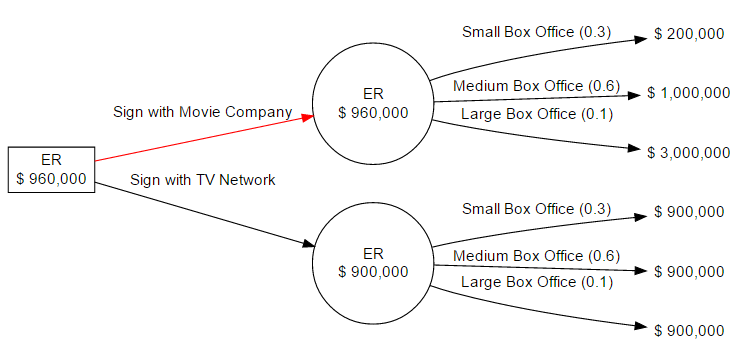# Bubble Chart (visualization)

In this example, we will replicate Mike Bostock’s bubble example. See here for details: https://bl.ocks.org/mbostock/4063269.
We use Joe Cheng’s bubbles package. All of this is inspired by Timelyportfolio, the king of htmlwidgets.

You’ll learn how to convert a complex JSON into a data.frame, and how to use this to plot hierarchic visualizations.

The data represents the Flare class hierarchy, which is a code library for creating visualizations. The JSON is long, deeply nested, and complicated.

fileName <- system.file("extdata", "flare.json", package="data.tree")
flareJSON <- readChar(fileName, file.info(fileName)$size) cat(substr(flareJSON, 1, 300)) ## { ## "name": "flare", ## "children": [ ## { ## "name": "analytics", ## "children": [ ## { ## "name": "cluster", ## "children": [ ## {"name": "AgglomerativeCluster", "size": 3938}, ## {"name": "CommunityStructure", "size": 3812}, ## {"name": "HierarchicalCluster", "size": 6714}, So, let’s convert it into a data.tree structure: library(jsonlite) flareLoL <- fromJSON(file(fileName), simplifyDataFrame = FALSE ) flareTree <- as.Node(flareLoL, mode = "explicit", check = "no-warn") flareTree$attributesAll
##  "size"
print(flareTree, "size", limit = 30)
##                            levelName  size
## 1  flare                                NA
## 2   ¦--analytics                        NA
## 3   ¦   ¦--cluster                      NA
## 4   ¦   ¦   ¦--AgglomerativeCluster   3938
## 5   ¦   ¦   ¦--CommunityStructure     3812
## 6   ¦   ¦   ¦--HierarchicalCluster    6714
## 7   ¦   ¦   °--MergeEdge               743
## 8   ¦   ¦--graph                        NA
## 9   ¦   ¦   ¦--BetweennessCentrality  3534
## 10  ¦   ¦   ¦--LinkDistance           5731
## 11  ¦   ¦   ¦--MaxFlowMinCut          7840
## 12  ¦   ¦   ¦--ShortestPaths          5914
## 13  ¦   ¦   °--SpanningTree           3416
## 14  ¦   °--optimization                 NA
## 15  ¦       °--AspectRatioBanker      7074
## 16  ¦--animate                          NA
## 17  ¦   ¦--Easing                    17010
## 18  ¦   ¦--FunctionSequence           5842
## 19  ¦   ¦--interpolate                  NA
## 20  ¦   ¦   ¦--ArrayInterpolator      1983
## 21  ¦   ¦   ¦--ColorInterpolator      2047
## 22  ¦   ¦   ¦--DateInterpolator       1375
## 23  ¦   ¦   ¦--Interpolator           8746
## 24  ¦   ¦   ¦--MatrixInterpolator     2202
## 25  ¦   ¦   ¦--NumberInterpolator     1382
## 26  ¦   ¦   ¦--ObjectInterpolator     1629
## 27  ¦   ¦   ¦--PointInterpolator      1675
## 28  ¦   ¦   °--RectangleInterpolator  2042
## 29  ¦   ¦--ISchedulable               1041
## 30  ¦   °--... 8 nodes w/ 0 sub         NA
## 31  °--... 8 nodes w/ 215 sub           NA

Finally, we can convert it into a data.frame. The ToDataFrameTable only converts leafs, but inherits attributes from ancestors:

flare_df <- ToDataFrameTable(flareTree,
className = function(x) x$parent$name,
packageName = "name",
"size")
head(flare_df)
##   className           packageName size
## 1   cluster  AgglomerativeCluster 3938
## 2   cluster    CommunityStructure 3812
## 3   cluster   HierarchicalCluster 6714
## 4   cluster             MergeEdge  743
## 5     graph BetweennessCentrality 3534
## 6     graph          LinkDistance 5731

This does not look spectacular. But take a look at this stack overflow question to see how people struggle to do this type of operation.

Here, it was particularly simple, because the underlying JSON structure is regular. If it were not (e.g. some nodes contain different attributes than others), the conversion from JSON to data.tree would still work. And then, as a second step, we could modify the data.tree structure before converting it into a data.frame. For example, we could use Prune and Remove to remove unwanted nodes, use Set to remove or add default values, etc.

## Plot

What follows has nothing to do with data.tree anymore. We simply provide the bubble chart printing for your enjoyment. In order to run it yourself, you need to install the bubbles package from github:

devtools::install_github("jcheng5/bubbles@6724e43f5e")
library(scales)
library(bubbles)
library(RColorBrewer)
bubbles(
flare_df$size, substr(flare_df$packageName, 1, 2),
tooltip = flare_df$packageName, color = col_factor( brewer.pal(9,"Set1"), factor(flare_df$className)
)(flare_df\$className),
height = 800,
width = 800
)